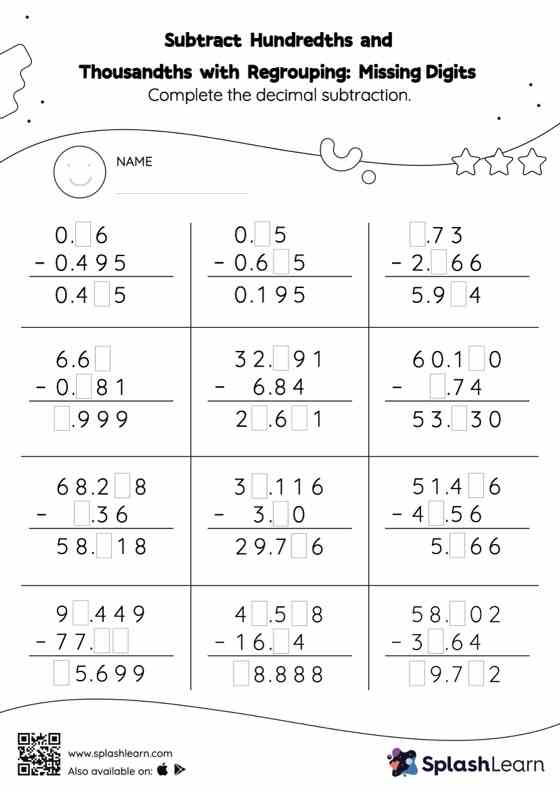# Subtract Hundredths and Thousandths with Regrouping: Missing Digits Worksheet

Home > Subtract Hundredths and Thousandths with Regrouping: Missing DigitsCan your young mathematician subtract hundredths and thousandths with regrouping? That's exactly what they do here. While subtracting decimals, students use the relationship between addition and subtraction to find the missing number. They also need to regroup numbers in subtract hundredths and thousandths with regrouping worksheet utilizing the relationship between ones, tenth, hundredth, thousandth, etc., To get to the result. As the worksheet uses the column method, it is helpful in getting students toward higher accuracy, especially with bigger numbers and in scenarios where regrouping is required.